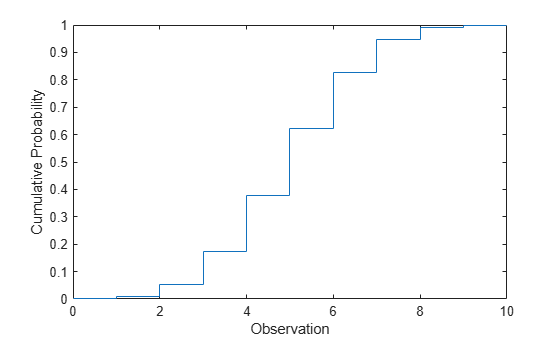## Binomial Distribution

### Overview

The binomial distribution is a two-parameter family of curves. The binomial distribution is used to model the total number of successes in a fixed number of independent trials that have the same probability of success, such as modeling the probability of a given number of heads in ten flips of a fair coin.

Statistics and Machine Learning Toolbox™ offers several ways to work with the binomial distribution.

### Parameters

The binomial distribution uses the following parameters.

ParameterDescriptionSupport
NNumber of trialsPositive integer
pProbability of success in a single trial$0\le p\le 1$

The sum of two binomial random variables that both have the same parameter p is also a binomial random variable with N equal to the sum of the number of trials.

### Probability Density Function

The probability density function (pdf) of the binomial distribution is

`$f\left(x|N,p\right)=\left(\begin{array}{c}N\\ x\end{array}\right){p}^{x}{\left(1-p\right)}^{N-x}\text{ };\text{ }x=0,1,2,...,N\text{\hspace{0.17em}},$`

where x is the number of successes in N trials of a Bernoulli process with the probability of success p. The result is the probability of exactly x successes in N trials. For discrete distributions, the pdf is also known as the probability mass function (pmf).

For an example, see Compute Binomial Distribution pdf.

### Cumulative Distribution Function

The cumulative distribution function (cdf) of the binomial distribution is

`$F\left(x|N,p\right)=\sum _{i=0}^{x}\left(\begin{array}{c}N\\ i\end{array}\right){p}^{i}{\left(1-p\right)}^{N-i}\text{ };\text{ }x=0,1,2,...,N\text{\hspace{0.17em}},$`

where x is the number of successes in N trials of a Bernoulli process with the probability of success p. The result is the probability of at most x successes in N trials.

For an example, see Compute Binomial Distribution cdf.

### Descriptive Statistics

The mean of the binomial distribution is Np.

The variance of the binomial distribution is Np(1 – p).

### Example

#### Fit Binomial Distribution to Data

Generate a binomial random number that counts the number of successes in `100` trials with the probability of success `0.9` in each trial.

`x = binornd(100,0.9)`
```x = 85 ```

Fit a binomial distribution to data using `fitdist`.

`pd = fitdist(x,'Binomial','NTrials',100)`
```pd = BinomialDistribution Binomial distribution N = 100 p = 0.85 [0.764692, 0.913546] ```

`fitdist` returns a `BinomialDistribution` object. The interval next to `p` is the 95% confidence interval estimating `p`.

Estimate the parameter `p` using the distribution functions.

`[phat,pci] = binofit(x,100) % Distribution-specific function`
```phat = 0.8500 ```
```pci = 1×2 0.7647 0.9135 ```
`[phat2,pci2] = mle(x,'distribution','Binomial',"NTrials",100) % Generic distribution function`
```phat2 = 0.8500 ```
```pci2 = 2×1 0.7647 0.9135 ```

#### Compute Binomial Distribution pdf

Compute the pdf of the binomial distribution with `10` trials and the probability of success `0.5`.

```x = 0:10; y = binopdf(x,10,0.5);```

Plot the pdf with bars of width `1`.

```figure bar(x,y,1) xlabel('Observation') ylabel('Probability')```#### Compute Binomial Distribution cdf

Compute the cdf of the binomial distribution with `10` trials and the probability of success `0.5`.

```x = 0:10; y = binocdf(x,10,0.5);```

Plot the cdf.

```figure stairs(x,y) xlabel('Observation') ylabel('Cumulative Probability')```#### Compare Binomial and Normal Distribution pdfs

When `N` is large, the binomial distribution with parameters `N` and `p` can be approximated by the normal distribution with mean N`*p` and variance N`*p*(1 ``p)` provided that p is not too large or too small.

Compute the pdf of the binomial distribution counting the number of successes in `50` trials with the probability `0.6` in a single trial .

```N = 50; p = 0.6; x1 = 0:N; y1 = binopdf(x1,N,p);```

Compute the pdf of the corresponding normal distribution.

```mu = N*p; sigma = sqrt(N*p*(1-p)); x2 = 0:0.1:N; y2 = normpdf(x2,mu,sigma);```

Plot the pdfs on the same axis.

```figure bar(x1,y1,1) hold on plot(x2,y2,'LineWidth',2) xlabel('Observation') ylabel('Probability') title('Binomial and Normal pdfs') legend('Binomial Distribution','Normal Distribution','location','northwest') hold off```The pdf of the normal distribution closely approximates the pdf of the binomial distribution.

#### Compare Binomial and Poisson Distribution pdfs

When `p` is small, the binomial distribution with parameters `N` and `p` can be approximated by the Poisson distribution with mean `N*p`, provided that `N*p` is also small.

Compute the pdf of the binomial distribution counting the number of successes in `20` trials with the probability of success `0.05` in a single trial.

```N = 20; p = 0.05; x = 0:N; y1 = binopdf(x,N,p);```

Compute the pdf of the corresponding Poisson distribution.

```mu = N*p; y2 = poisspdf(x,mu);```

Plot the pdfs on the same axis.

```figure bar(x,[y1; y2]) xlabel('Observation') ylabel('Probability') title('Binomial and Poisson pdfs') legend('Binomial Distribution','Poisson Distribution','location','northeast')```The pdf of the Poisson distribution closely approximates the pdf of the binomial distribution.

### Related Distributions

• Bernoulli Distribution — The Bernoulli distribution is a one-parameter discrete distribution that models the success of a single trial, and occurs as a binomial distribution with N = 1.

• Multinomial Distribution — The multinomial distribution is a discrete distribution that generalizes the binomial distribution when each trial has more than two possible outcomes.

• Normal Distribution — The normal distribution is a two-parameter continuous distribution that has parameters μ (mean) and σ (standard deviation). As N increases, the binomial distribution can be approximated by a normal distribution with µ = Np and σ2 = Np(1 – p). See Compare Binomial and Normal Distribution pdfs.

• Poisson Distribution — The Poisson distribution is a one-parameter discrete distribution that takes nonnegative integer values. The parameter λ is both the mean and the variance of the distribution. The Poisson distribution is the limiting case of a binomial distribution where N approaches infinity and p goes to zero while Np = λ. See Compare Binomial and Poisson Distribution pdfs.

 Abramowitz, Milton, and Irene A. Stegun, eds. Handbook of Mathematical Functions: With Formulas, Graphs, and Mathematical Tables. 9. Dover print.; [Nachdr. der Ausg. von 1972]. Dover Books on Mathematics. New York, NY: Dover Publ, 2013.

 Evans, Merran, Nicholas Hastings, and Brian Peacock. Statistical Distributions. 2nd ed. New York: J. Wiley, 1993.

 Loader, Catherine. Fast and Accurate Computation of Binomial Probabilities. July 9, 2000.